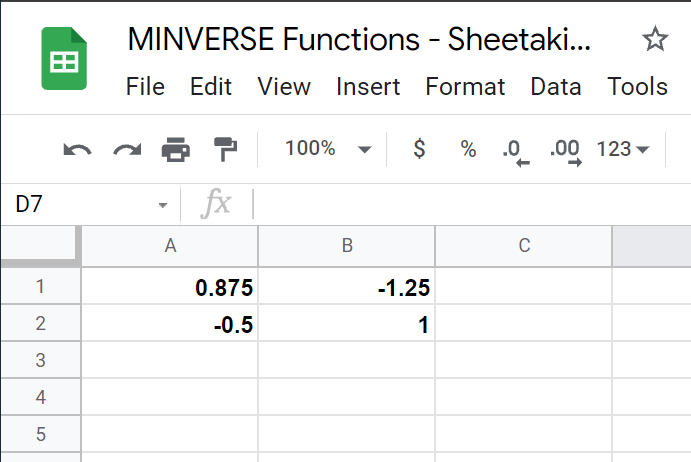# How to Use MINVERSE Function in Google Sheets

The MINVERSE function in Google Sheets is useful when you need to compute the multiplicative inverse of a square matrix.

The square matrix can refer to either a given array or range in the worksheet representing the matrix to be computed.

The rules for using the `MINVERSE` function in Google Sheets are as follows:

• The function just requires one argument, the square matrix. It may come in the form of an array (e.g., [1,4,2,5]) or as a range (“A2:B4”).
• The function then outputs the multiplicative inverse of the matrix as a range.
• The multiplicative inverse of the matrix refers to a matrix that when multiplied to the original matrix produces an identity matrix.
• The identity matrix is a square matrix with ones on the main diagonal and zeros elsewhere. Later, we’ll be showing an example to illustrate this property more clearly.

Let’s begin with a quick demonstration.

A group of tourists took a trip to an amusement park. They first rode the carousel, which priced tickets depending on age: \$4 for adults and \$2 for children. Overall, they spent \$122 dollars. Afterwards, the tourists took the Ferris wheel, which cost \$5 for adults and 3\$ for children. All the Ferris wheel tickets cost the group \$163.5. Given this, how many adults and children were in this tourist group?

Using matrix multiplication and the multiplicative inverse we can solve this equation. Later in this guide, we’ll be showing the `MINVERSE` function in action as we try to solve the problem above. We’ll also be needing the MMULT function since that will allow us to perform matrix multiplication later.

For now, let’s learn how to write the `MINVERSE` function ourselves in Google Sheets!

## The Anatomy of the MINVERSE Function

So the syntax (the way we write) the DAYS function is as follows:

```=MINVERSE(square_matrix)
```

Let’s dissect this thing and understand what each of these terms means:

• = the equal sign is how we start any function in Google Sheets.
• MINVERSE() is our `MINVERSE` function. It outputs the multiplicative inverse of the given square_matrix
• square_matrix refers to the range or array we would like to find the inverse of.  It must have an equal number of rows and columns.
• The square matrix must be written properly as an array. For example, a 2×2 matrix where the top row is {2,3} and bottom row is {5,4} must be written as such: {2,3};{5,4}

## A Real Example of Using MINVERSE Function

Let’s look into an example of the `MINVERSE` function being used in a Google Sheet spreadsheet.In the spreadsheet above, we have two unknown values, the number of adults and children. Mathematically, if we were to multiply the matrix of people (in blue) with the matrix of prices (in red), the product should correspond with the final sum (in purple).

Formally, we can write this as `Tourists*Prices = Sums`. To isolate just Tourists, we must multiply the inverse of the Prices matrix with the Sums matrix.To get the values in the range D5:E6, we just need to use the following formula in cell D5:

``` =MINVERSE(A5:B6)
```

Afterward, we can use the MMULT function to get the product of the inverse we got and the SUMS matrix, as seen below.Using the `MMULT` and `MINVERSE` functions, we now know that there were 25 adults and 11 children on the trip.

You can make a copy of the spreadsheet above using the link I have attached below.

If you’re ready to play around with the `MINVERSE` function in Google Sheets, let’s try it out step-by-step!

## How to Use MINVERSE Function in Google Sheets

In this guide, let’s try doing the same computation above, but now with arrays instead of a cell range as input.

1. To start using the `MINVERSE` function, select the cell we will first put our function’s output. In this example, we’ll start with cell A1.
2. Next, we must type the equal sign ‘=‘ to begin our function, followed by ‘MINVERSE(‘, the name of our function.
3. A tooltip box appears with info on the `MINVERSE` function. We may click on the arrow on the top-right hand corner of the box to minimize the pop-up if necessary.4. Next, we must type the arguments of our function. In this case, MINVERSE only needs one argument, the square matrix. Given the same price problem earlier, we can write the price matrix like so:5. Afterward, simply hit Enter on your keyboard to let the function evaluate. If we followed all the steps correctly, the `MINVERSE` formula should output the following matrix in our worksheet!This step-by-step guide shows how easy it is to find the multiplicative inverse of a square matrix! You can now use the `MINVERSE` functions in Google Sheets together with the various other Google Sheets formulas available to help you set up powerful and useful spreadsheets!

Do subscribe to our newsletter to be the first to know about the latest Google Sheets guides and tutorials from us.Our goal this year is to create lots of rich, bite-sized tutorials for Google Sheets users like you. If you liked this one, you'll love what we are working on! Readers receive ✨ early access ✨ to new content.

##### You May Also Like## How to Use XOR Function in Google Sheets

The XOR function in Google Sheets lets you check if the data you input in the spreadsheet meets…## How to Use REGEXMATCH Function in Google Sheets

The REGEXMATCH Function in Google Sheets is useful if you want to know if a piece of text…## How to Use DAYS Function in Google Sheets

The DAYS function in Google Sheets is useful when you need to know the number of days between…## How To Use IMCSC Function in Google Sheets

The IMCSC function in Google Sheets is useful when you need to return the cosecant of a particular…